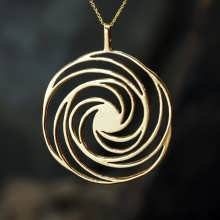# Spirale d’Or or

### Apporte la beauté et la paix dans votre vie au travers de l'harmonie avec la nature

The Golden Mean Spiral
The Golden Mean Spiral structure represents two well known sacred geometry patterns: the golden mean (phi) spiral and the Fibonacci spiral. The Mysterious Golden Mean also known as the "Golden Ratio" or Phi value (1.618) after the 21st letter of the Greek alphabet, PHI [φ] is a pattern that appears all throughout nature and the universe. It is a cosmic law which is even more mysterious and profound in its implications than Pi. Like Pi, Phi is a number with no arithmetical solution. The decimals just keep on going to infinity without ever repeating themselves. The unique thing about this number is that it can be found incorporated in most organic structures. From the bone structure of human beings to the seed pattern of a sunflower to the spiral of a sea shell, the phi proportion is there, underlying all biological structures, seems to be a geometrical blueprint for life itself.

Plato went so far as to call the phi ratio the "key to the physics of the cosmos". Phi is calculated at 1.6180339+..., and while it cannot be worked out arithmetically, it can be easily obtained with a compass and straightedge.

Golden mean in Pyramids
The phi ratio is found in the architecture of the Great Pyramid in the triangle formed by the height, half-base, and the apothem, or diagonal. In other words, the basic cross-section of the structure demonstrates the Golden Section. The Golden Section shows up again and again in Giza and in much more baffling and tedious ways.

The Fibonacci Spiral
Another Sacred Geometry Spiral Pattern can be found in the Fibonacci sequence.
which bears a special relationship to phi, and to the pyramids at Giza. First postulated by the medieval mathematician Leonardo Fibonacci, this sequence was used to describe the growth patterns of plants. It goes: 1,1,2,3,5,8,13,21,34,55,89,144,233, etc. It is made by adding the last two numbers of the sequence to get the next one, as in: 1+1=2, 1+2=3, 2+3=5, 3+5=8, 5+8=13, etc. This sequence's relationship to phi is that if you divide one term of the sequence into the next one, the result begins to quickly approach the transcendental number 1.6180339+... Of course, you can never actually get to it exactly, since it has no mathematical solution, it just keeps getting closer and closer, to infinity. Aerial photographs show that the pyramids at Giza are positioned in such a way that they fall on a line which accurately defines a Fibonacci spiral. The spiral passes exactly through the centers of each pyramid.

Even the microscopic realm is not immune to Fibonacci. The DNA molecule measures 34 angstroms long by 21 angstroms wide for each full cycle of its double helix spiral. These numbers, 34 and 21, are numbers in the Fibonacci series, and their ratio 1.6190476 closely approximates Phi, 1.6180339.

Scientists have found that the height of one unit of the DNA helix is related to its width in a proportion equal to the "The Golden Spiral (Ratio)" or the Fibonacci and Padovan number series that form the bridge from the number patterns found in in the double helix of DNA and a cross-sectional view of the DNA molecule from the top reveals two pentagons (whose angles are determined by the golden ratio).

So once again we can see that we are the universe itself trying to learn itself and understand itself through countless eons of evolution…

The pendant is a symbol of Harmony and beauty.